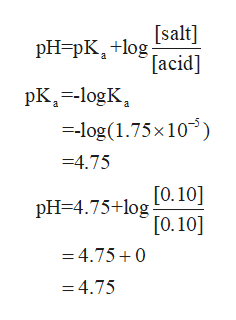# ANSWER B,C & DThe Ka value of acetic (ethanoic) acid is 1.75x 10-5 .a) Calculate the pH of a 0.10M solution of CH3COOH(aq).b) 50mL of 0.10M of acetic acid and 50mL of 0.10M of sodium acetate are mixed. What is the pH of the resulting solution? Include any assumptions made.c) 0.0025 mole of NaOH is added to 100mL of the solution in b). What is now the pH of the resulting solution? Assume that volume of the solution does not change when NaOH is added.d) Comment on the effectiveness of the solution in b) as a buffer giving a reason. How could a more effective buffer solution be prepared?

Question
107 views

The Ka value of acetic (ethanoic) acid is 1.75x 10-5 .

a) Calculate the pH of a 0.10M solution of CH3COOH(aq).

b) 50mL of 0.10M of acetic acid and 50mL of 0.10M of sodium acetate are mixed. What is the pH of the resulting solution? Include any assumptions made.

c) 0.0025 mole of NaOH is added to 100mL of the solution in b). What is now the pH of the resulting solution? Assume that volume of the solution does not change when NaOH is added.

d) Comment on the effectiveness of the solution in b) as a buffer giving a reason. How could a more effective buffer solution be prepared?

check_circle

Step 1

According to the Henderson equation,help_outlineImage TranscriptionclosepH=pK, +log [salt] [acid] pK-logK -log(1.75x10 a 4.75 [0.10] pH 4.75+log [0.10] =4.75+0 =4.75 fullscreen
Step 2

When 0.0025 moles of NaOH are added to the 100ml of the solution

Step 3

This basically adds 0.025M of Na ions and it decreases the acid...

### Want to see the full answer?

See Solution

#### Want to see this answer and more?

Solutions are written by subject experts who are available 24/7. Questions are typically answered within 1 hour.*

See Solution
*Response times may vary by subject and question.
Tagged in

### Equilibrium Concepts